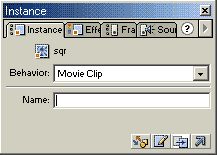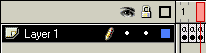# Flash Script – Matrix Text Effect

You’ve seen the film The Matrix, and now you’d like to create the falling green text effect from the credits? No problem! Download the sample files here.

1. Create a text field as shown below.Give it a variable name of "text".

2. Next, convert this to a movie clip, and name it "text".

3. Select the existing movieclip, press f8, and convert it once again to a movie clip.

4. Give it an instance name of "matrix".5. Now, go into the time line of movie clip text, and create a new layer above the existing layer. Name it "actions".

6. Create two blank key frames in the actions layer. In the first frame, insert:

``````text = random(2)+"r"+random(2)+"r"+random(2)+"r"+random(2)+"r"+random(2)+"r"+random(2)
+"r"+random(2)+"r"+random(2)+"r"+random(2);``````

7. In the second frame, insert this action:

``gotoAndPlay (1);``

8. Now, go to the main movie time line, and go into the time line of the "matrix" movie clip.

9. Create three keyframes in this layer, and insert the following action into the first key frame:``````mov = 0;
ran = getProperty("", _x);``````

10. In the second key frame, insert:

``````setProperty ("", _y, mov=mov+2);
snowy = getProperty("", _y);
setProperty ("", _x, (ran+random(2)));
if(snowy > 310)
{
setProperty ("", _y, 50);
mov=0;
ran = ran - 10;
}``````

11. In the third key frame insert:

``gotoAndPlay (2);``

12. Now, go back to the main timeline, and create two key frames in this layer.

13. Insert this action into the first key frame:

``````if (matrixparticles<150)
{
``gotoAndPlay (2);``